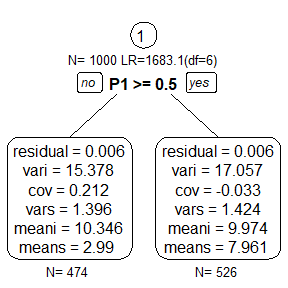# Getting Started with the semtree package

library(semtree)
library(OpenMx)

## Simulate data

set.seed(23)
N <- 1000
M <- 5
icept <- rnorm(N, 10, sd = 4)
slope <- rnorm(N, 3, sd = 1.2)
p1 <- sample(c(0,1),size=N,replace=TRUE)
growth.data <- data.frame(x,p1)
names(growth.data) <- c(paste0("X",1:M),"P1")

## Specify an OpenMx model

manifests <- names(growth.data)[1:5]
growthCurveModel <- mxModel("Linear Growth Curve Model Path Specification",
type="RAM",
manifestVars=manifests,
latentVars=c("intercept","slope"),
mxData(growth.data, type="raw"),
# residual variances
mxPath(
from=manifests,
arrows=2,
free=TRUE,
values = c(.1, .1, .1, .1, .1),
labels=c("residual","residual","residual","residual","residual")
),
# latent variances and covariance
mxPath(
from=c("intercept","slope"),
arrows=2,
connect="unique.pairs",
free=TRUE,
values=c(2, 0, 1),
labels=c("vari", "cov", "vars")
),
mxPath(
from="intercept",
to=manifests,
arrows=1,
free=FALSE,
values=c(1, 1, 1, 1, 1)
),
mxPath(
from="slope",
to=manifests,
arrows=1,
free=FALSE,
values=c(0, 1, 2, 3, 4)
),
# manifest means
mxPath(
from="one",
to=manifests,
arrows=1,
free=FALSE,
values=c(0, 0, 0, 0, 0)
),
# latent means
mxPath(
from="one",
to=c("intercept", "slope"),
arrows=1,
free=TRUE,
values=c(1, 1),
labels=c("meani", "means")
)
) # close model

## Run a tree

tree <- semtree(model = growthCurveModel, data = growth.data)
#> <U+2716> Variable P1 is numeric but has only few unique values. Consider recoding as ordered factor.
#> <U+2714> Tree construction finished [took 2s].

## Plotting

plot(tree)